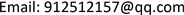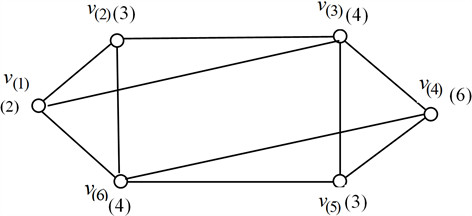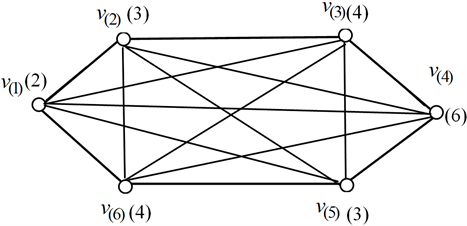﻿ 基于蚁群算法的赋权图点覆盖问题 Vertex Covering Problem of Weighted Graphs Based on Ant Colony Algorithm

Vol.06 No.09(2017), Article ID:23095,7 pages
10.12677/AAM.2017.69136

Vertex Covering Problem of Weighted Graphs Based on Ant Colony Algorithm

Peiwen Wu, Jingrong Chen, Luye Ji

School of Mathematics and Physics, Lanzhou Jiaotong University, Lanzhou GansuReceived: Nov. 27th, 2017; accepted: Dec. 14th, 2017; published: Dec. 21st, 2017ABSTRACT

Point covering problem is a NP complete problem in real life. Ant colony algorithm is a new bionic stochastic optimization algorithm emerging in recent years. In this paper, the minimum coverage problem of weighting graphs is studied by using the ant colony algorithm. An approximate algorithm based on ant colony algorithm is proposed to solve the minimum point covering problem. By modifying the state transition probability formula of ants and simplifying the state transition rules, the corresponding mathematical model is established, and then the approximate algorithm for solving the point coverage is obtained. Finally, an example is analyzed. The experimental results show that the algorithm is effective.

Keywords:Point Coverage, Ant Colony Algorithm, State Transition Rule, Weighted Graph1. 引言

Avis等设计了几个算法，其整数规划的近似比为2，匹配算法的近似比为2，贪心算法的近似比为 $\sqrt{\Delta }/2+3/2$ ，其中 $\Delta$ 为图的最大度  。Delbot等改进了文献  中的算法，并证明所得算法的近似比都不大于文献  的结果  。最小点覆盖问题的参数化算法在1993年被Buss等第一次提出，其时间复杂性为 $O\left(kn+{2}^{k}{k}^{2k+2}\right)$  。点覆盖问题的智能化算法是Khuri等在1994年提出的，其将最小加权点覆盖问题看成一个有约束的组合优化问题并且引入了遗传算法进行求解  。Stefan等采用禁忌搜索和模拟退火相结合的混合算法来研究最小权点覆盖问题，其结果优于很多启发式逼近算法  。周康等在闭环DNA算法中通过删除实验得到了最小点覆盖的补集，通过求其补集从而得到其最小点覆盖  。吕健康等根据顶

2. 蚁群算法解决最小点覆盖问题的基本原理

${\eta }_{jk}=\frac{\sum _{\left(r,j\right)\in {E}_{c}}{\psi }_{k}\left(r,j\right)}{\omega \left(j\right)}$ (1)

${p}_{j}^{k}=\left\{\begin{array}{l}1,q>{q}_{0},j={\mathrm{max}}_{i\in {A}_{k}}\left\{{\tau }_{i}{\eta }_{ik}^{\beta }\right\}\\ 0,q>{q}_{0},j\ne {\mathrm{max}}_{i\in {A}_{k}}\left\{{\tau }_{i}{\eta }_{ik}^{\beta }\right\}\\ \frac{{\tau }_{j}{\eta }_{jk}^{\beta }}{\sum _{r\in {A}_{K}}{\tau }_{j}{\eta }_{jk}^{\beta }},q\le {q}_{0}\end{array}$ (2)

${\tau }_{i}=\left\{\begin{array}{l}\left(1-\rho \right){\tau }_{i}+\rho \Delta {\tau }_{i},i\in {v}^{\prime }\\ \left(1-\rho \right){\tau }_{i},\text{otherwise}\end{array}$ (3)

$\Delta {\tau }_{i}=\left\{\begin{array}{l}0,i\notin {V}^{\prime }\\ \frac{1}{\sum _{j\in {V}^{\prime }}\omega \left(j\right)},i\in {V}^{\prime }\end{array}$ (4)

$\rho \in \left(0,1\right)$ 为一个模拟信息素强度蒸发率的参数。

${\tau }_{i}=\left(1-\phi \right){\tau }_{i}+\phi {\tau }_{0}$ (5)

${\tau }_{0}$ 为信息素放在每个顶点的初始值。参数 $\phi$ 是规定的特殊局部更新规则的值。

3. 算法设计

Step1：定义连接函数 ${\psi }_{k}$

${\psi }_{k}\left(i,j\right)=\left\{\begin{array}{l}1,\left(i,j\right)\in E\\ 0,\left(i,j\right)\in {E}_{c}-E\end{array}$

Step2：若蚂蚁 $k$ 访问了 ${E}_{c}$ 中的某个点，则与该点相连的边连接值修改为0。即： ${\psi }_{k}\left(i,j\right)=0$

Step3：计算每一点的动态启发因子 ${\eta }_{jk}$

${\eta }_{jk}=\frac{\sum _{\left(r,j\right)\in {E}_{c}}{\psi }_{k}\left(r,j\right)}{\omega \left(j\right)}$

Step4：对局部信息素进行更新：

${\tau }_{i}=\left(1-\phi \right){\tau }_{i}+\phi {\tau }_{0}$

Step5：由状态转移公式计算出蚂蚁 $k$ 选择下一顶点的概率：

${p}_{j}^{k}=\left\{\begin{array}{l}1,j={\mathrm{max}}_{i\in {A}_{K}}\left\{{\tau }_{i}{\eta }_{jk}^{\beta }\right\}\\ 0,j\ne {\mathrm{max}}_{i\in {A}_{K}}\left\{{\tau }_{i}{\eta }_{jk}^{\beta }\right\}\end{array}$

Step6：当完成一次环游时对全局信息素进行更新，更新函数：

${\tau }_{i}=\left\{\begin{array}{l}\left(1-\rho \right){\tau }_{i}+\rho \Delta {\tau }_{i},i\in {v}^{\prime }\\ \left(1-\rho \right){\tau }_{i},\text{otherwise}\end{array}$

4. 算法实例

Step1：由连接函数 ${\psi }_{k}\left(i,j\right)=\left\{\begin{array}{l}1,\left(i,j\right)\in E\\ 0,\left(i,j\right)\in {E}_{c}-E\end{array}$ 给定各边的连接，蚂蚁1以 ${v}_{1}$ 为出发点，有 ${S}_{1}=\left\{{v}_{1}\right\}$

Step2：用连接函数 ${\psi }_{k}\left(i,j\right)=0$ 更新与点 ${v}_{1}$ 关联边的连接值；

Step3：计算 ${\tau }_{i}{\eta }_{jk}^{\beta }$ ，有 ${\tau }_{i}{\eta }_{jk}^{\beta }\ne 0$ 转Step4；

Step4：计算 $\mathrm{max}\left\{{\tau }_{i}{\eta }_{jk}^{\beta }\right\}=0.001$$j=3$ ，将其并入集合 ${S}_{1}$${S}_{1}=\left\{{v}_{1},{v}_{3}\right\}$ ，转Step2；

Step2：用连接函数 ${\psi }_{k}\left(i,j\right)=0$ 更新与点 ${v}_{3}$ 关联边的连接值；

Step3：进行局部信息素更新，计算 ${\tau }_{i}{\eta }_{jk}^{\beta }$ ，有 ${\tau }_{i}{\eta }_{jk}^{\beta }\ne 0$ 转Step4；

Step4：计算 $\mathrm{max}\left\{{\tau }_{i}{\eta }_{jk}^{\beta }\right\}=0.002$$j=6$ ，将其并入集合 ${S}_{1}$${S}_{1}=\left\{{v}_{1},{v}_{2},{v}_{6}\right\}$ ，转Step2；

Step2：用连接函数 ${\psi }_{k}\left(i,j\right)=0$ 更新与点 ${v}_{6}$ 关联边的连接值；

Step3：进行局部信息素更新，计算 ${\tau }_{i}{\eta }_{jk}^{\beta }$ ，有 ${\tau }_{i}{\eta }_{jk}^{\beta }\ne 0$ 转Step4；

Step4：计算 $\mathrm{max}\left\{{\tau }_{i}{\eta }_{jk}^{\beta }\right\}=0.004$$j=5$ ，将其并入集合 ${S}_{1}$${S}_{1}=\left\{{v}_{1},{v}_{3},{v}_{6},{v}_{5}\right\}$ ，转Step2；

Step2：用连接函数 ${\psi }_{k}\left(i,j\right)=0$ 更新与点 ${v}_{5}$ 关联边的连接值；

Step3：进行局部信息素更新，计算 ${\tau }_{i}{\eta }_{jk}^{\beta }$ ，有 ${\tau }_{i}{\eta }_{jk}^{\beta }=0$ ，输出 ${S}_{1}=\left\{{v}_{1},{v}_{3},{v}_{5},{v}_{6}\right\}$

Step5：计算 $|{S}_{1}|=\underset{j\in {S}_{1}}{\mathrm{min}}\omega \left(j\right)=13$ ，算法停止。

Step6：一次环游完成，对全局信息素进行更新；

Step7：计算 $S=\mathrm{min}\left\{{S}_{1},{S}_{2},\cdots ,{S}_{k},\cdots ,{S}_{n}\right\}$ ，输出最小点覆盖集 ${S}_{2}=\left\{{v}_{1},{v}_{2},{v}_{5},{v}_{3}\right\}$

5. 结束语Figure 1. Original diagramFigure 2. Complete diagram

Vertex Covering Problem of Weighted Graphs Based on Ant Colony Algorithm[J]. 应用数学进展, 2017, 06(09): 1119-1125. http://dx.doi.org/10.12677/AAM.2017.69136

1. 1. Johnson, D.S. (1974) Approximation Algorithms for Combinatorial Problems. JCSS, 9, 256-278.

2. 2. Karp, R.M. (1972) Reducibility among Combinatorial Problems. Plenum Press, New York, 85-100.
https://doi.org/10.1007/978-1-4684-2001-2_9

3. 3. Garey, M.R. and Andjohnson, D. (1979) Computers and In-tractability. Freeman and Co., New York.

4. 4. Savage, C. (1982) Depth-First Search and the Vertex Cover Problem. Information Processing Letters, 14, 233-235.
https://doi.org/10.1016/0020-0190(82)90022-9

5. 5. Pitt, L. (1985) A Simple Probabilistic Approximation Algo-rithm for Vertex Cover. Department of Computer Science, Yale University, New Haven.

6. 6. Avis, D. and Imamura, T. (2007) A List Heuristic for Vertex Cover. Operations Research Letters, 35, 201-204.
https://doi.org/10.1016/j.orl.2006.03.014

7. 7. Delbot, F. and Andlaforest, C. (2008) A Better List Heuristic for Vertex Cover. Information Processing Letters, 107, 125-127.
https://doi.org/10.1016/j.ipl.2008.02.004

8. 8. Buss, J.F. and Goldsmith, J. (1993) Nondeterminism within P. Springer Berlin Heidelberg, 480(3): 348-359.
https://doi.org/10.1137/0222038

9. 9. Khuri, S. and Back, T. (1994) An Evolutionary Heuristic for the Minimum Vertex Cover Problem. Genetic Algorithms within the Framework of Evolutionary Computation, 86-90.

10. 10. Voß, S. and Fink, A. (2012) A Hybridized Tabu Search Approach for the Minimum Weight Vertex Cover Problem. Journal of Heuristics, 18, 869-876.
https://doi.org/10.1007/s10732-012-9211-9

11. 11. 周康, 许进. 最小顶点覆盖问题的闭环DNA算法[J]. 计算机工程与应用, 2006, 20(9): 7-9.

12. 12. 吕健康, 张国基. 求一般图的最小顶点覆盖集问题的混合贪婪算法[J]. 科学技术与工程, 2010, 20(10): 4891-4895.

13. 13. 郑光勇, 李肯立, 潘果, 徐雨明. 化学反应优化算法求解最小顶点覆盖问题[J]. 小型微型计算机系统, 2015, 36(2): 301-305.

14. 14. Dorigo, M., Maniezzo, V. and Colorni, A. (1996) Ant System: Optimization by a Colony of Cooperating Agents. IEEE Transaction on Systems, Man, and Cybernetics-Part B, 26, 29-41.
https://doi.org/10.1109/3477.484436

15. 15. 陈京荣, 俞建宁, 李引珍. 基于蚁群算法的多属性路径选择模型[J]. 系统工程, 2009, 27(5): 30-34.

16. 16. 陈烨. 带杂交算子的蚁群算法[J]. 计算机工程, 2001, 27(12): 74-76, 126.

17. 17. 何小峰, 马良 求解图着色问题的量子蚁群算法[J]. 运筹学学报, 2013, 2(17): 19-26.

18. 18. Shyu, S.J., Yin, P.-Y. and Lin, B.M.T. (2004) An Ant Colony Optimization Algorithm for the Minimum Weight Vertex Cover Problem. Annals of Operations Research, 131, 283-304.
https://doi.org/10.1023/B:ANOR.0000039523.95673.33